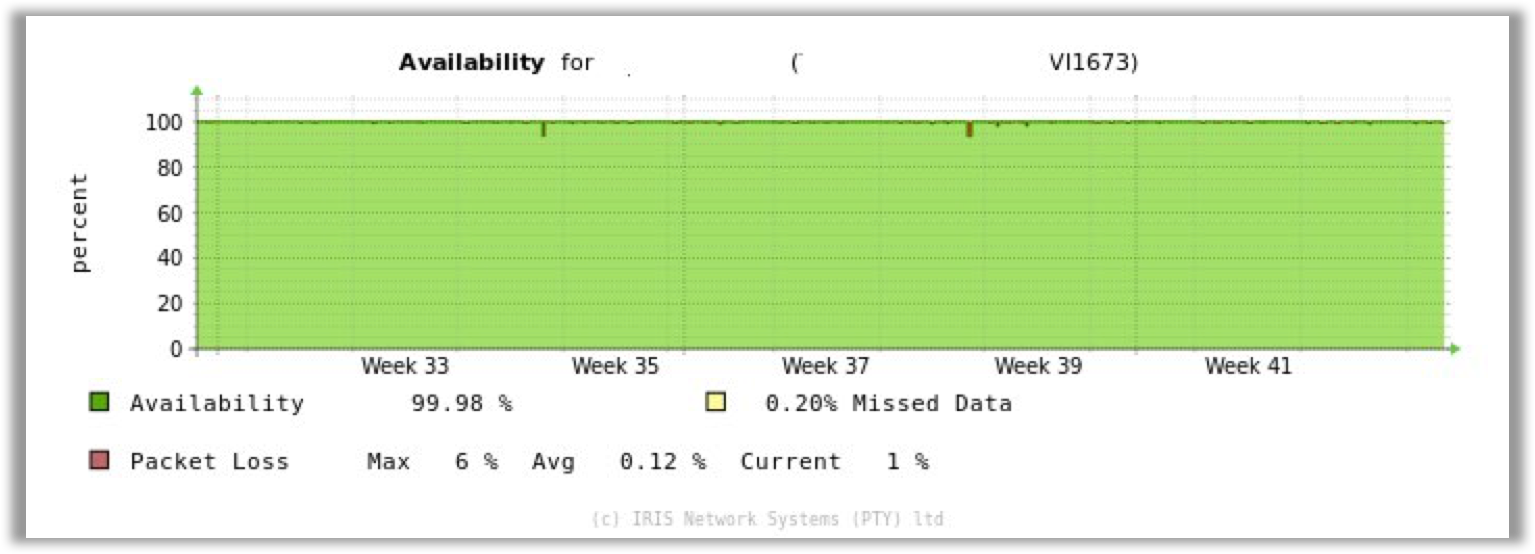As per graph availability, over a week is 100%, however in the report, the availability is 99.79% and 97.66%. Which one is the correct value?

This is puzzling, so let's have a look at it in more detail.

If you are pulling 24 hours worth of data and see an outage on a link, then pull the same information on the same size graph for a month that outage that was displayed on the 24 hour graph disappears on the month graph, here is why:

On some occasions you might be looking at a graph for 24 hours, and in those 24 hours there was an outage of a few hours.Then when you pull a month's graph the outage seems to disappear.The reason for this is that the default graph is plotted at 400 pixels, when pulling 24 hours worth of data thats (24*12) 288 data points as we poll every 5 minutes, to fit 288 data points onto a 400 pixel wide graph is very doable and therefore we can show quite a bit of detail.

If you then look at a months worth of data on the same graph size ( 400px ) we now need to fit 8928 ( 31*288 ) pixels on that graph (as we keep 60 days worth of 5-minute data ). So in order to fit 8928 datapoints on a 400 pixel graph we need to make some decisions around what we display and roll datapoints into pixels, so the data then looks averaged and the outage then appears to "disappear" off the graph.

In this case the amount of percentage that had dropped was minute that when calculated the average would have turned out as 100%. As the report does not do the averaging the data seems to be more raw.